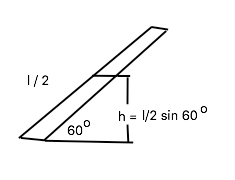# A uniform rod of mass 6.0 kg and length 2.2 m is free to rotate about one end. If the rod is...

## Question:

A uniform rod of mass 6.0 kg and length 2.2 m is free to rotate about one end. If the rod is released from rest at an angle of 60 degrees with respect to the horizontal, what is the speed (in m/s) of the tip of the rod as it passes the horizontal position?

## Conservation of Energy

The formula for the conservation of energy is given by;

{eq}KE_{initial} + PE_{initial} = KE_{final} + PE_{final} \\ \ \\ \frac{1}{2} m v^2_{initial} + mgh_{initial} = \frac{1}{2} m v^2_{final} + mgh_{final} \\ \ \\ {/eq}

Wherein,

{eq}v_{initial} \ and \ v_{final} \ {/eq} is the initial and final velocity in the system.

The Rotational kinetic Energy is given by the formula,

{eq}KE \ = \dfrac 12 I \omega^2 {/eq}

Wherein,

"I" is the moment of inertia of the rigid body involve or undergoing rotation and

{eq}\omega {/eq} is the angular speed of the body.

## Answer and Explanation:

Applying energy conservation,

Change in potential energy = change in kinetic energy

{eq}mgh = \dfrac 12 I \omega^2 \\ {/eq}

Consider the figure,Here, we can see the height h is equal to,

{eq}h = \dfrac l2 sin 60^{\circ} \ = \ \dfrac {\sqrt{3}}{4} l \\ \rm{and} \\ \rm{ moment \ of \ inertia } \ I \ \rm{is \ equal \ to} \\ I = \dfrac 13 ml^2 {/eq}

then we have,

{eq}mgh = \dfrac 12 I \omega^2 \\ mg \dfrac {\sqrt{3}}{4} l = \dfrac 12 \times \dfrac 13 ml^2 \times \omega^2 \\ \\ \omega^2 = \dfrac {3g \sqrt{3}}{2l} \\ \ = \dfrac {3(9.8) \sqrt{3}}{2l} \\ \omega^2 = 11..57324 \\ \omega = 3.401 \dfrac {rad}{s} \\ \\ \\ \rm{since} \\ v \ = \ \omega R \\ \ = \ \omega l \\ \ = \ 3.401 \dfrac {rad}{s} (2.2) \\ v \ = \ 7.4842 \dfrac ms {/eq}